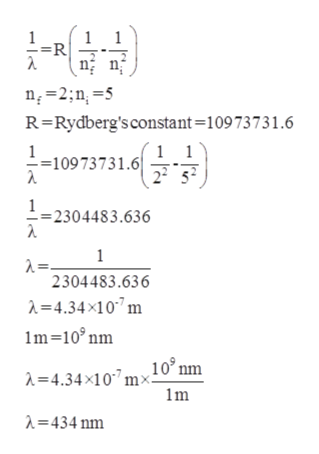# use the bohr equation to find the wavelength of the photon emitted when an H atom undergoes a transition from n=5 to n=2

Question
6 views

use the bohr equation to find the wavelength of the photon emitted when an H atom undergoes a transition from n=5 to n=2

check_circle

Step 1

The Bohr’s equation for finding the wavelength of photon emitted is similar to the Rydberg equation.  The equation is

Step 2

The given transition is from n=5 to n=2.  ...help_outlineImage Transcriptionclose1 =R n 2;n5 R-Rydberg's constant=10973731.6 1 1 2252 =10973731.6 1 2304483.636 1 2=. 2304483.636 4.34x10 m m 10 nm A =4.34x10mx10°nm m 434 nm fullscreen

### Want to see the full answer?

See Solution

#### Want to see this answer and more?

Solutions are written by subject experts who are available 24/7. Questions are typically answered within 1 hour.*

See Solution
*Response times may vary by subject and question.
Tagged in

### Chemistry